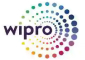New update is available. Click here to update.
Topics

# Contains Duplicate ll

Moderate0/80
Average time to solve is 35m+1 more companies

## Problem statement

Given an array 'arr' of 'N' integers and an integer 'K'. The array 'arr' may contain duplicate integers. Return "true" if the array contains any duplicate element within the 'K' distance from each other, otherwise, return "false".

Detailed explanation ( Input/output format, Notes, Images )
Constraints :
``````1 <= T <= 10
1 <= N <= 10^5
1 <= K <= 10^5
-10^9 <= arr[i] <= 10^9

Time Limit: 1sec
``````
Sample Input 1 :
``````1
5 2
3 4 9 4 2
``````
Sample Output 1 :
``````true
``````
Explanation For Sample Output 1 :
``````From index 1 to 3 there are two 4’s.
``````
Sample Input 2 :
``````1
10 4
3 2 0 -4 7 -9 -8 10 5 -1
``````
Sample Output 2 :
``````false
``````
Explanation For Sample Output 2 :
``````There is no duplicate element within a distance of 4 in the given array .
``````Console